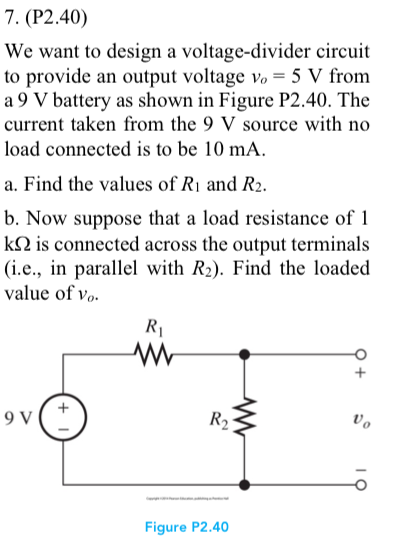### Create an Account

Home / Questions / 7. (P2.40) We want to design a voltage-divider circuit to provide an output voltage vo = 5 V from a

# 7. (P2.40) We want to design a voltage-divider circuit to provide an output voltage vo = 5 V from a7. (P2.40) We want to design a voltage-divider circuit to provide an output voltage vo = 5 V from a 9 V battery as shown in Figure P2.40. The current taken from the 9 V source with no load connected is to be 10 mA a. Find the values of Rı and R2. b. Now suppose that a load resistance of 1 k is connected across the output terminals (i.e., in parallel with R2). Find the loaded value of vo R2 9 V Figure P2.40

Apr 01 2020 View more View LessSubscribe To Get Solution